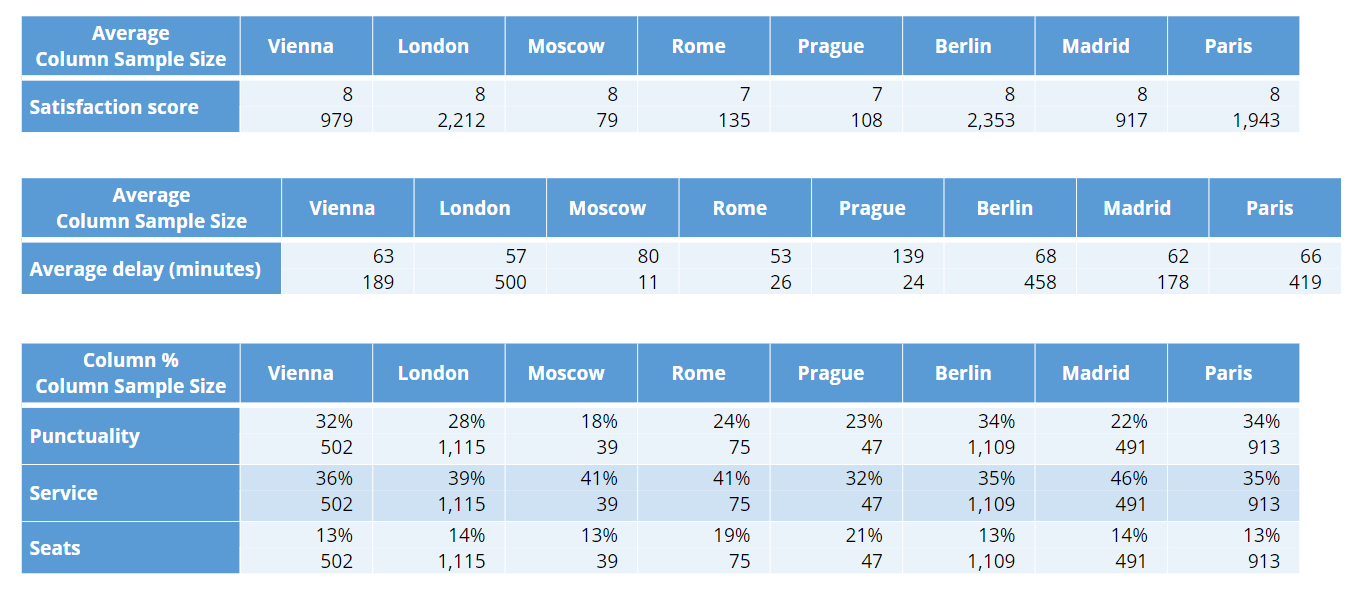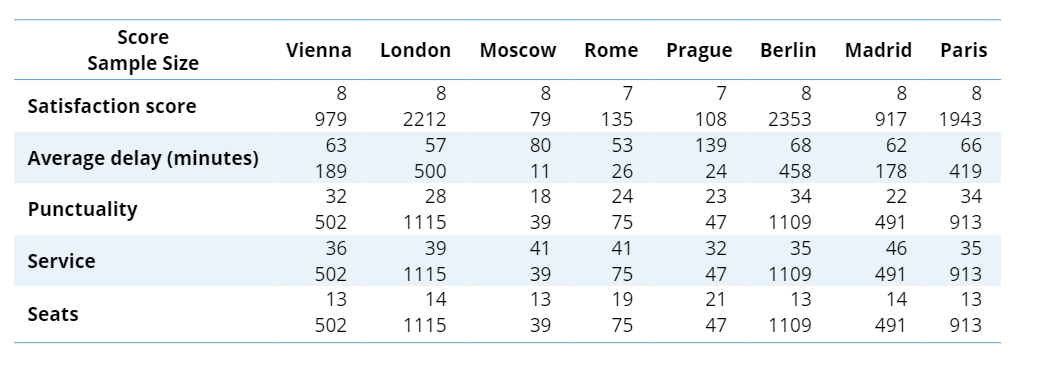Want to share your content on R-bloggers? click here if you have a blog, or here if you don't.

You can use R to to splice and dice tables that have been created with Displayr’s built-in statistical engine. This is particularly handy when you want to mix results from numeric data with those for categorical data in the same table, or when combining results into a single table would otherwise require wrangling the data. When you show a single statistic on each table, you can use standard methods for combining tables in R. This is discussed in our other post, How to Build a Brand Funnel in Displayr using R. If, on the other hand, your table contains multiple statistics, including the Column Comparisons statistical tests, a different approach is required. In this post I show you how to combine more complicated tables, and provide some more examples of how Displayr tables work in R.

## Creating tables

In this post I am concerned with tables that have been created using Display’s built-in statistical engine. To use this, you must first add a data set using Home > Data Set. Then, such a table is created by clicking-and-dragging a variable set from under the Data Sets section in the bottom left, or by clicking Insert > Table, and using the Row and Column drop-down menus in the Object Inspector on the right of the screen to select the data. The statistics shown in the table can be changed using Inputs > STATISTICS > Cells, also in the Object Inspector.

In this post I consider a set of tables from a survey for a bus service in Europe. The tables tell us information about key results pertaining to trips departing from different cities.I want to create a summary of all the key results in a single topline table for the start of my report. Importantly, people in the survey were asked different things, and so the sample size varies. It is important that I report the sample sizes in the same table as my topline figures.

We will use R to combine these tables together.

## Simple tables

Tables which show a single statistic are easier to deal with. In this case, such tables are seen in R as matrices. A matrix is just a set of values spread across rows and columns. All the values in a matrix must be of the same type.  The standard R functions rbind and cbind can be used to combine these tables. For an example of how to combine matrices in Displayr, see How to Build a Brand Funnel in Displayr using R.

## Combining tables with multiple statistics

Tables which include multiple statistics, like the ones I want to combine, are no longer matrices. This is because each “row” of the table is actually two rows, one for the main statistic and one for the sample size. Instead, each of these tables is an object known as an array. You can think of an array as two matrices stacked on top of one another, along a third dimension.To combine a set of tables like the ones from the first section above above, you can use the following method.

1. Select Insert > R Output.
2. Paste the lines of code below into the R CODE section.
3. Change the names of the tables you are referring to in the second line. To find the name of a table, select the table on your page, and then look in Properties > GENERAL > Name in the Object Inspector on the right.
4. Tick the Automatic box. This ensures that whenever the input data changes, the new table will update accordingly.

The code required is:

```library(abind)
new.table = abind(table.satisfaction, table.delay, table.favorite, along = 1)
dimnames(new.table)[] = c("Score","Sample Size")
new.table

```

The resulting table is:There are several key points to notice about the code above:

• I use the abind() function from the abind library.
• I listed the reference names of the tables I wanted to combine.
• I used  the along argument to specify which dimension of the array to join the tables along. The dimension ‘1’ stands for the rows. If I wanted to combine columns, I would instead supply dimension ‘2’.
• I set dimnames(new.table)[] to change the names of the statistics, as they appear in the top left corner.

To set the table styling, I have used Appearance > Table Styles.

So remember, when you need to combine many tables which show multiple statistics, use abind!

Get started in Displayr, or if you want to learn more, check out our other handy How To guides!